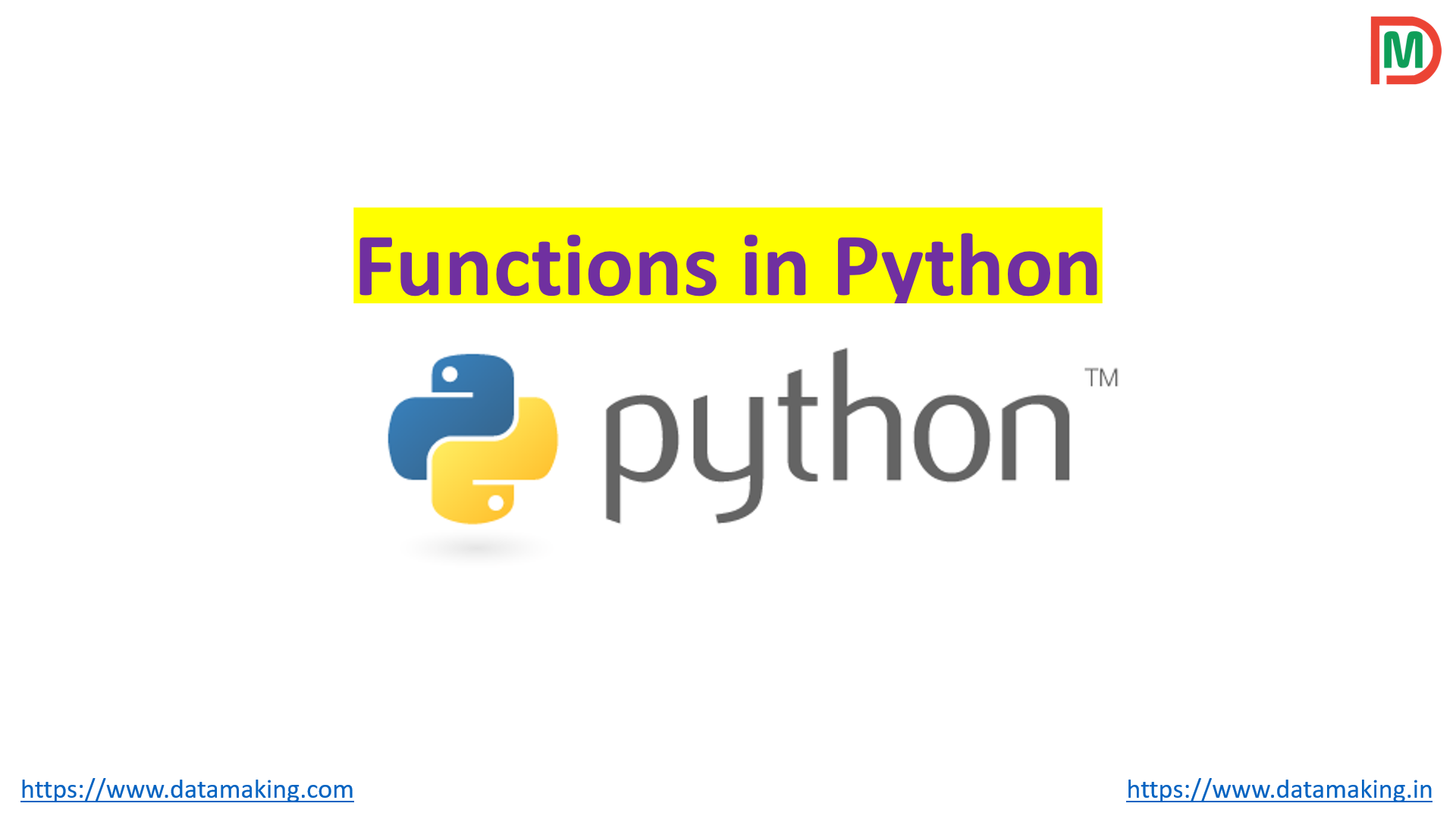## Functions in Python

A function is a block of code which only runs when it is called. You can pass data, known as parameters or arguments, into a function. A function can return data as a result.

In Python, a function is defined using the def keyword. To call a function, use the function name followed by parenthesis. You need to take care of indentation in Python.

``````def hello():
print('Hello World!')

# call the function
hello()

print('Outside function')``````

### Arguments

Information can be passed into functions as arguments. Arguments are specified after the function name, inside the parentheses. You can add as many arguments as you want, just separate them with a comma.

The following example has a function with one argument (fname). When the function is called, we pass along a first name, which is used inside the function to print the full name:

``````def my_function(fname):
print(fname + " Refsnes")

my_function("Emil")
my_function("Tobias")
my_function("Linus")``````